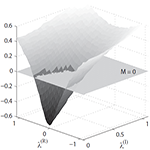Published/Posted: July 5, 2011

Authors: Cohen, A. B.

Abstract: The subject of this thesis is the exploration of chaotic synchronization for novel applications including time-series prediction and sensing. We begin by characterizing the nonlinear dynamics of an optoelectronic time-delayed feedback loop. We show that synchronization of an accurate numerical model to experimental measurements provides a way to assimilate data and forecast the future of deterministic chaotic behavior. Next, we implement an adaptive control method that maintains isochronal synchrony for a network of coupled feedback loops when the interaction strengths are unknown and time-varying. Control signals are used as real-time estimates of the variations present within the coupling paths. We analyze the stability of synchronous solutions for arbitrary coupling topologies via a modified master stability function that incorporates the adaptation response dynamics. Finally, we show that the master stability function, which is derived from a set of linearized equations, can also be experimentally measured using a two-node network, and it can be applied to predict the convergence behavior of large networks.

Citation:
A. B. Cohen, "Synchronization and prediction of chaotic dynamics on networks of optoelectronic oscillators", PhD Physics, University of Maryland (2011)

Manuscript: Cohen_PhD_Thesis_2011.pdf